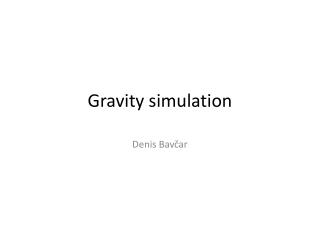DownloadDownload PresentationGravity simulation

# Gravity simulation

Download Presentation## Gravity simulation

- - - - - - - - - - - - - - - - - - - - - - - - - - - E N D - - - - - - - - - - - - - - - - - - - - - - - - - - -
##### Presentation Transcript

1. Gravitysimulation Denis Bavčar

2. Gravity in 2D • 2 large objects which attract other objects • N small objects with mass low enoughthat their influence on other objects can be ignored. • Equation in original c++ code: p.vx += 0.03 * p1.m / (d*d) * (p1.x - p.x)/d; • 0.03 = a constant that replaces G (gravitational constant)in the program • p1.m = mass of the other object • d = distance between two objects • (p1.x – p.x)/d = turns 2D acceleration into horizontal acceleration

3. Theimportant part of .c code

4. Kernel